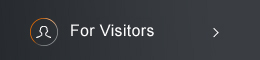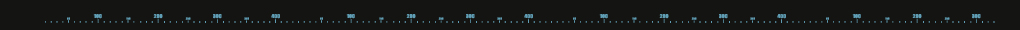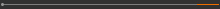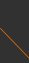Sponsored by[ Events ]

﻿
Activity Search
Sort out
 select all Seminars and Talks Conference and Workshop Lectures and Courses ElseField
 select all Number Theory and Representation Theory Algebraic Geometry Differential Geometry and Geometric Analysis Differential Equations and Stochastic Analysis Scientific Computing Interdisciplinary Studies Laboratory of Data Science Harmonic Analysis OthersYear
 select 2015 2016 2017 2018 2019 2020 2021 2022 2023Taipei Postdoc Seminar14:00 - 15:00, May 18, 2022 (Wednesday) Cisco Webex, Online seminar (線上演講 Cisco Webex) Introduction to the Optimal Transportation Problem and the Monge-Ampere Equation Seonghyeon Jeong (NCTS) Abract Since the problem was formulated by G. Monge in 18C, there was not a lot of progress on the optimal transportation problem until L. Kantorovich found the relaxed problem. After L. Kantorovich, there was a lot of progress in the optimal transportation problem by many mathematicians. One of the important discoveries is that the solution of the optimal transportation problem is very closely related to a PDE called the Monge-Ampere equation. Therefore, studying some properties of the solutions to the optimal transportation problem (for example, regularity of the solution) became studying the Monge-Ampere equation. In this talk, I will talk about the formulation of the optimal transportation problem, Kantorovich's work, and the relation to the Monge-Ampere equation. To simplify some arguments, the cost function of the optimal transportation, we will use the distance square cost function. If time permits, I will introduce some regularity results in the Monge-Ampere equation. Link information Meeting number (access code): 2512 882 9887 Meeting password: x4xJUAkjG32(C) 2021 National Center for Theoretical Sciences